Area of Parallelograms 1
(10 Questions)
(see Standards below...)

From Mr. Anker Tests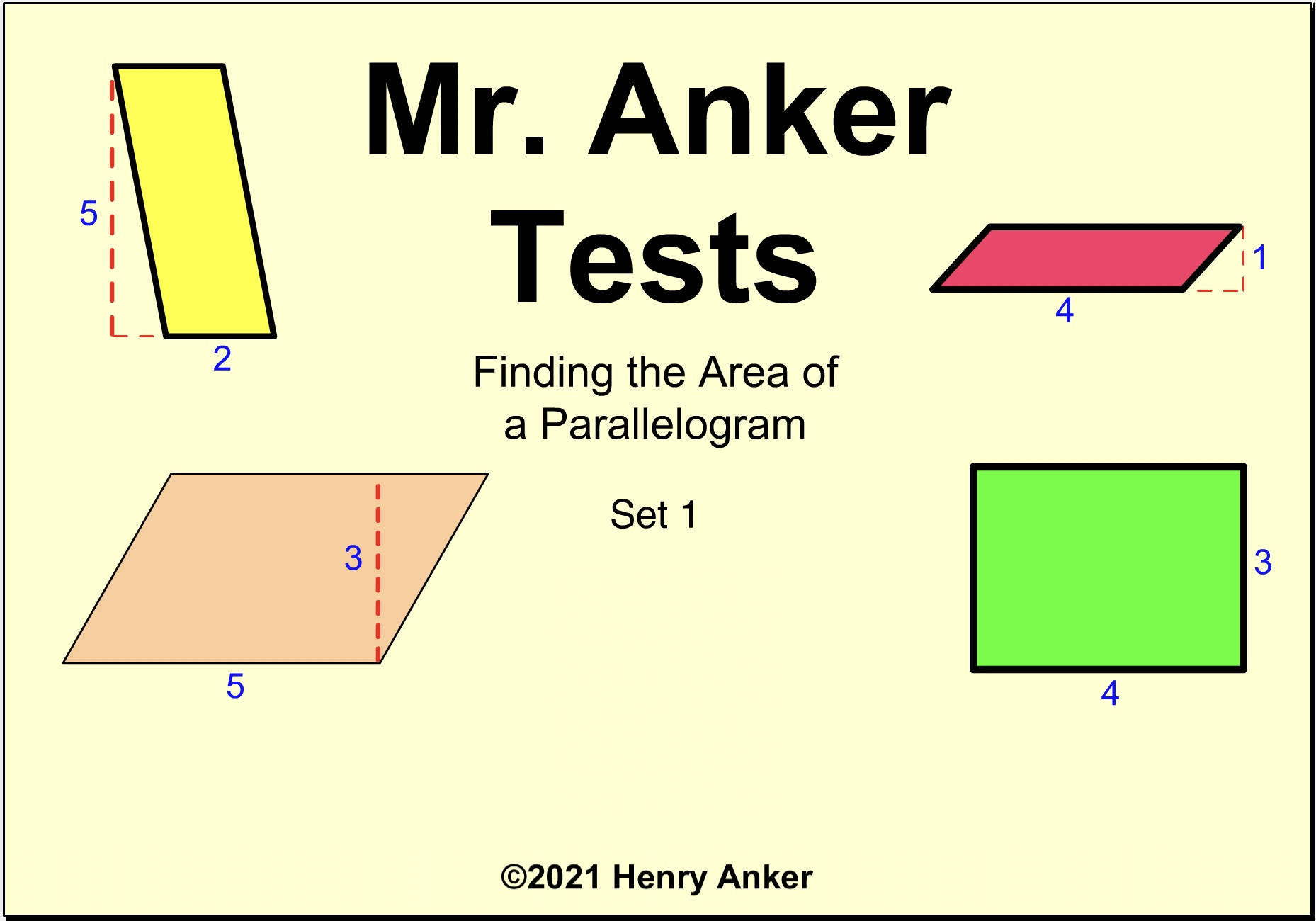Area of Parallelograms Standards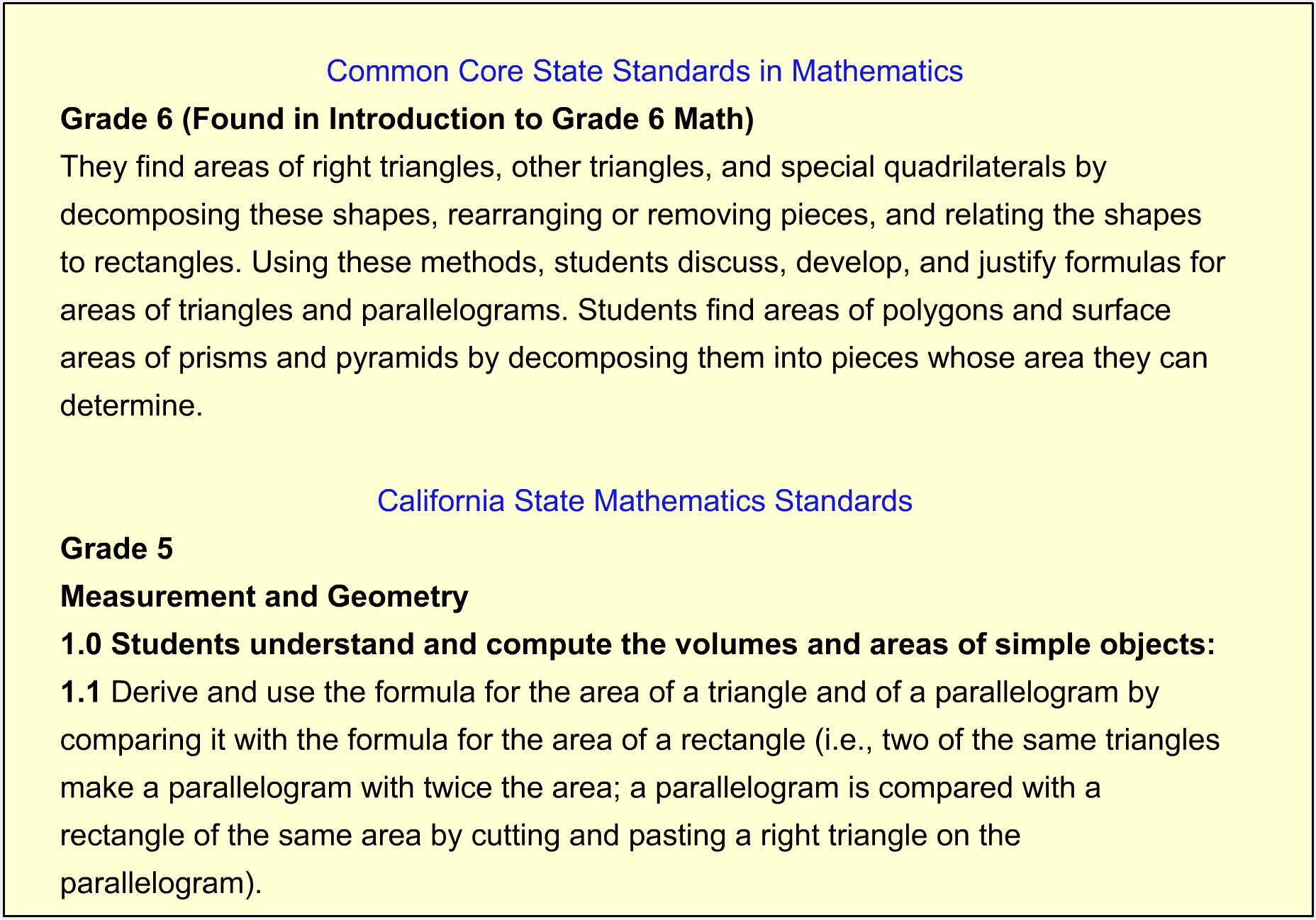Area of Parallelograms Help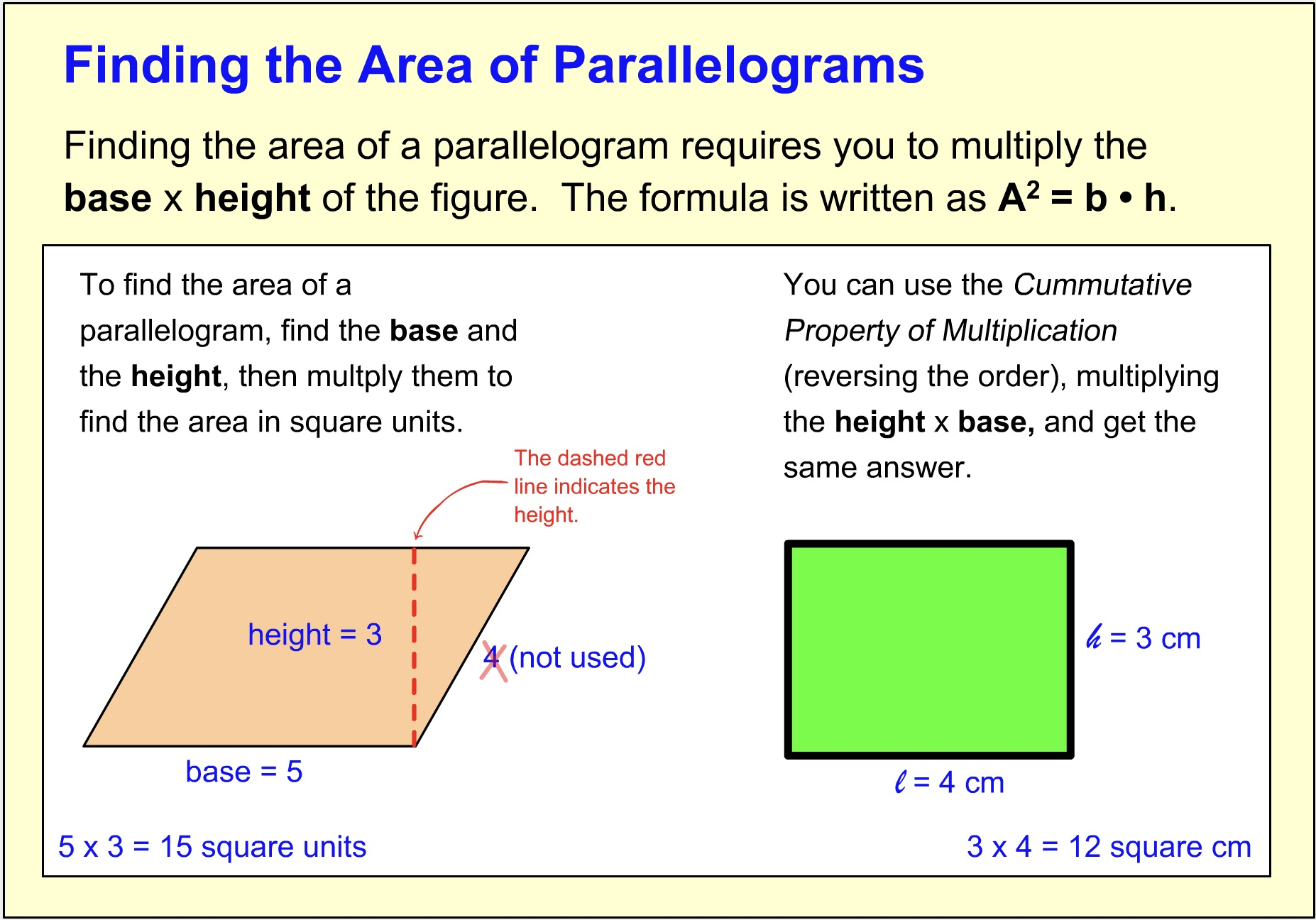1. What is the area, in square units, of the parallelogram? *
1 point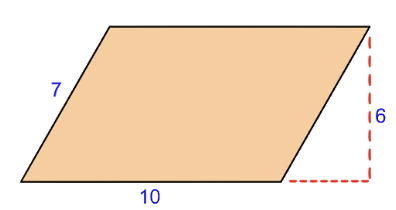2. What is the area, in square units, of the parallelogram? *
1 point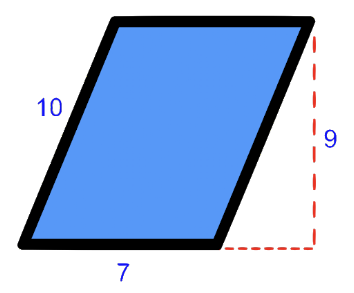3. What is the area, in square units, of the parallelogram? *
1 point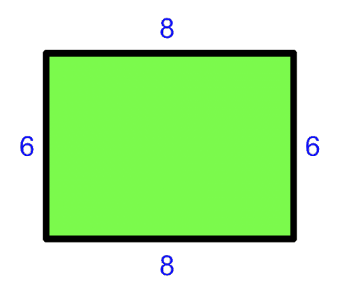4. What is the area, in square units, of the parallelogram? *
1 point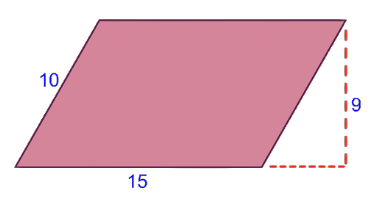5. Which equation below shows the correct way to find the area of a parallelogram? *
1 point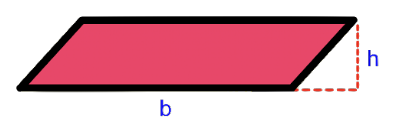6. What is the area, in square units, of the parallelogram? *
1 point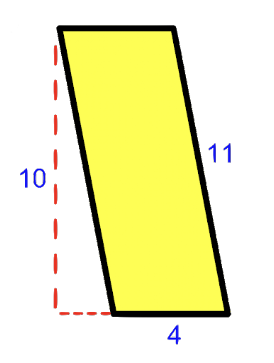7.  Enter the area, in square centimeters, of this parallelogram, in the space below (just the numbers). *
1 point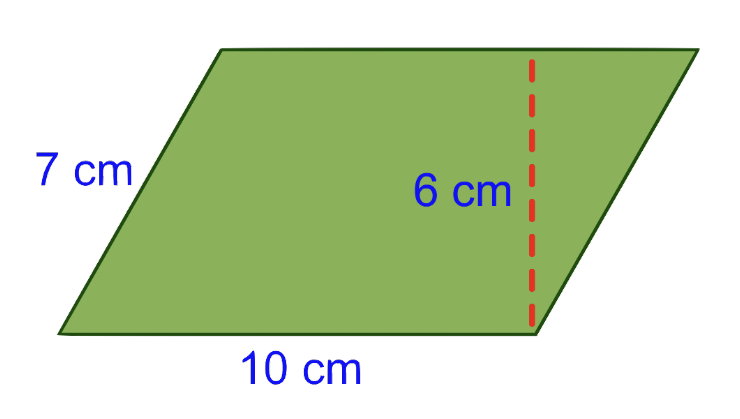8. Enter the area, in square centimeters, of this parallelogram, in the space below (just the numbers). *
1 point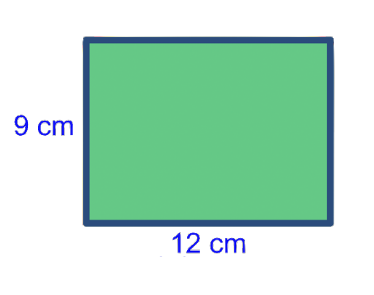9.  Enter the area, in square centimeters, of this parallelogram, in the space below (just the numbers). *
1 point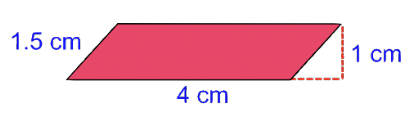10. Enter the area, in square centimeters, of this parallelogram, in the space below (just the numbers). *
1 point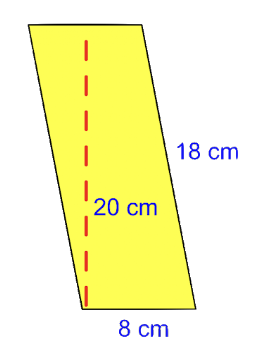Submit
Clear form